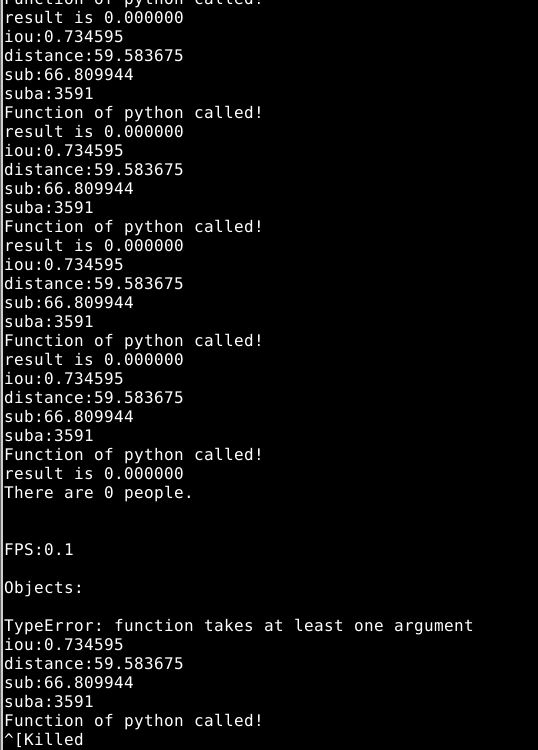srand((unsigned)time(NULL));

``````for (i=0;i<50;i++){

io = rand()/(double)(RAND_MAX);
printf("iou:%f\n",io);

dist = rand()/(double)(RAND_MAX/100);
printf("distance:%f\n",dist);

su = rand()/(double)(RAND_MAX/100);
printf("sub:%f\n",su);

sua = rand()%50000;
printf("suba:%d\n",sua);
Py_Initialize();
if(!Py_IsInitialized())
{
printf("Python init failed!\n");
return;
}
PyRun_SimpleString("import sys");
PyRun_SimpleString("sys.path.append('//home//wangxiangsheng//wuhao//test')");

PyObject *pName = NULL;
PyObject *pModule = NULL;
PyObject *pDict = NULL;
PyObject *pFunc = NULL;
PyObject *pArgs = NULL;
PyObject *pResult = NULL;
pName = PyString_FromString("RF");
pModule = PyImport_Import(pName);
pDict = PyModule_GetDict(pModule);
pFunc = PyDict_GetItemString(pDict,"randomF");

pArgs = Py_BuildValue("(f,f,f,i)",io,dist,su,sua);

pResult = PyObject_CallObject(pFunc,pArgs);
PyArg_Parse(pResult, "i", &res);
printf("result is %d\n",res);
``````
`````` 调用的python脚本：RF.py

``````

import sklearn as skl
from sklearn.externals import joblib
from sklearn.ensemble import RandomForestClassifier

def randomF(iou,distance,sub,suba):

``````test_data = [[iou,distance,sub,suba]]

output = clf.predict(test_data)
print output
return output
````````````     Py_Initialize();
if(!Py_IsInitialized())
{
printf("Python init failed!\n");
return;
}
PyRun_SimpleString("import sys");
PyRun_SimpleString("sys.path.append('//home//wangxiangsheng//darknet//src')");

PyObject *pName = NULL;
PyObject *pModule = NULL;
PyObject *pDict = NULL;
PyObject *pFunc = NULL;
PyObject *pArgs = NULL;
PyObject *pResult = NULL;

pName = PyString_FromString("RF");
pModule = PyImport_Import(pName);
if(!pModule)
{
getchar();
}
pDict = PyModule_GetDict(pModule);
if(!pDict)
{
printf("Can't find dict in RF!\n");
}

pFunc = PyDict_GetItemString(pDict,"randomF");
if(!pFunc || !PyCallable_Check(pFunc))
{
printf("Can't find function!\n");
getchar();
}

for(j=0;j<10;j++){
for(w=j+1;w<39;w++){
float media = box_iou(para[j].p, para[w].p);
float iout;
if (media<=0){
iout = 0.000000;
} else {
iout = media;
}
int p,suba;
float res;
suba = abs(para[j].barea-para[w].barea);
float sub=0;
for(p=0;p<64;p++){
sub = sub + pow(para[j].hist[p]-para[w].hist[p],2);
}
float distance;
distance = sqrt(pow(para[w].p.x-para[j].p.x,2)+pow(para[w].p.y-para[j].p.y,2));
printf("%f-%f-%f-%d \n",iout,distance,sub,suba);
pArgs = Py_BuildValue("(f,f,f,i)",iout,distance,sub,suba);
pResult = PyObject_CallObject(pFunc,pArgs);
PyArg_Parse(pResult, "f", &res);

printf("result is %f\n",res);
``````

1个回答TLedTS emmm。。。我就是参考了这些网站改的，只调用一次的话没有问题，但是重复调用就不能重复Py_Finalize，扔掉这个之后反倒能正常运行。我试验了一个小demo能正常运行我写的程序，就是在嵌入yolo源码中时除了问题。c语言调用python脚本

python脚本调用c函数

php调用C语言程序

Java 调用C语言程序
Java 调用C语言程序 参考java核心技术 卷2 高级特性 第9版 第12章 P793 Linux环境下： #1.compile java javac HelloNative.java #2.generate .h javah HelloNative #3.generate dynamic link library gcc -fPIC -I /opt
matiab调用C语言程序

c程序调用python脚本，python脚本中的危险分子global变量

C 语言程序调用Fortran 语言程序的方法与实例
C 语言程序调用Fortran 语言程序的方法与实例 才漪
c语言程序c语言程序

shell中调用python脚本

PHP文件中调用Python脚本

Shell脚本中调用python脚本
/usr/local/bin/python2.6 xxx.py [参数] 或 python xxx.py [参数]
c 调用Python脚本和函数（一）
1.py import numpy as np def data(): # matrix=np.arange(1,100) matrix=[[0,1,2,3],[1,1,1,1]] print matrix 1.cpp #include&lt;Python.h&gt; int main() { Py_Initialize(); PyRun_SimpleString("...
CCS 中C语言程序调用线性汇编

C语言程序中的文件调用详解

VB.net中调用c语言程序计算问题

Matlab调用C语言程序简单实例
matlab的功能强大，不仅仅因为matlab的强大的函数库和验算本式的计算方式，更重要的是因为他有强大的接口。 今天，我才抄着写出了一个自己的运行在matlab中的C程序，是书上原来的例子。但是，知道可以调用的惊奇还是十分令人愉快的。
delphi如何调用c语言程序？？？

C语言程序中的内存分配

C语言程序中的函数

VC中如何调用Python脚本？

Java程序中调用Python脚本的方法
&amp;#13; 在程序开发中，有时候需要Java程序中调用相关Python脚本，以下内容记录了先关步骤和可能出现问题的解决办法。&amp;#13; 1、在Eclipse中新建Maven工程；&amp;#13; 2、pom.xml文件中添加如下依赖包之后update maven工程；&amp;#13; 　　&amp;#13; &amp;#13; 　　　　　&amp;lt;dependency&amp;gt;&amp;#13; ...

c语言程序结果。c语言程序结果。c语言程序结果。c语言程序结果。
c语言程序结果。c语言程序结果。c语言程序结果。c语言程序结果。c语言程序结果。c语言程序结果。c语言程序结果。c语言程序结果。c语言程序结果。c语言程序结果。c语言程序结果。
C语言程序
C语言程序
c语言程序
agaffaafaf